xProbability amplitudeEncyclopedia
In quantum mechanics
Quantum mechanics
Quantum mechanics, also known as quantum physics or quantum theory, is a branch of physics providing a mathematical description of much of the dual particle-like and wave-like behavior and interactions of energy and matter. It departs from classical mechanics primarily at the atomic and subatomic...

, a probability amplitude is a complex number
Complex number
A complex number is a number consisting of a real part and an imaginary part. Complex numbers extend the idea of the one-dimensional number line to the two-dimensional complex plane by using the number line for the real part and adding a vertical axis to plot the imaginary part...

whose modulus
Absolute value
In mathematics, the absolute value |a| of a real number a is the numerical value of a without regard to its sign. So, for example, the absolute value of 3 is 3, and the absolute value of -3 is also 3...

squared represents a probability
Probability
Probability is ordinarily used to describe an attitude of mind towards some proposition of whose truth we arenot certain. The proposition of interest is usually of the form "Will a specific event occur?" The attitude of mind is of the form "How certain are we that the event will occur?" The...

or probability density
Probability density function
In probability theory, a probability density function , or density of a continuous random variable is a function that describes the relative likelihood for this random variable to occur at a given point. The probability for the random variable to fall within a particular region is given by the...

.
For example, if the probability amplitude of a quantum state is, the probability of measuring
Measurement in quantum mechanics
The framework of quantum mechanics requires a careful definition of measurement. The issue of measurement lies at the heart of the problem of the interpretation of quantum mechanics, for which there is currently no consensus....

that state is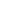. The values taken by a normalised wave function  at each point are probability amplitudes, since gives the probability density at position .

The principal use of probability amplitudes is as the physical meaning of the wavefunction, a link first proposed by Max Born
Max Born
Max Born was a German-born physicist and mathematician who was instrumental in the development of quantum mechanics. He also made contributions to solid-state physics and optics and supervised the work of a number of notable physicists in the 1920s and 30s...

and a pillar of the Copenhagen interpretation
Copenhagen interpretation
The Copenhagen interpretation is one of the earliest and most commonly taught interpretations of quantum mechanics. It holds that quantum mechanics does not yield a description of an objective reality but deals only with probabilities of observing, or measuring, various aspects of energy quanta,...

of quantum mechanics. In fact, the properties of the wave function were being used to make physical predictions (such as emissions from atoms being at certain discrete energies) before any physical interpretation was offered. Born was awarded half of the 1954 Nobel Prize in physics
Nobel Prize in Physics
The Nobel Prize in Physics is awarded once a year by the Royal Swedish Academy of Sciences. It is one of the five Nobel Prizes established by the will of Alfred Nobel in 1895 and awarded since 1901; the others are the Nobel Prize in Chemistry, Nobel Prize in Literature, Nobel Peace Prize, and...

for this understanding, though it was vigorously contested at the time by the original physicists working on the theory, such as Schrödinger and Einstein. Therefore, the probability thus calculated is sometimes called the "Born probability", and the relationship used to calculate probability from the wavefunction is sometimes called the Born rule
Born rule
The Born rule is a law of quantum mechanics which gives the probability that a measurement on a quantum system will yield a given result. It is named after its originator, the physicist Max Born. The Born rule is one of the key principles of quantum mechanics...

.

These probability amplitudes have special significance because they act in quantum mechanics as the equivalent of conventional probabilities, with many analogous laws. For example, in the classic double-slit experiment
Double-slit experiment
The double-slit experiment, sometimes called Young's experiment, is a demonstration that matter and energy can display characteristics of both waves and particles...

where electrons are fired randomly at two slits, an intuitive interpretation is that , where is the probability of that event. However, it is impossible to observe which slit is passed through without altering the electron. Thus, when not watching the electron, the particle cannot be said to go through either slit and this simplistic explanation does not work. However, the complex amplitudes taken by the two wavefunctions which represent the electron passing each slit do follow a law of precisely the form expected , and the calculations agree with experiment. This is the principle of quantum superposition
Quantum superposition
Quantum superposition is a fundamental principle of quantum mechanics. It holds that a physical system exists in all its particular, theoretically possible states simultaneously; but, when measured, it gives a result corresponding to only one of the possible configurations.Mathematically, it...

, and explains the requirement that amplitudes be complex, as a purely real formulation has too few dimensions to describe the system's state when superposition is taken into account.

## A basic example

Take a quantum system that can be in two possible states: for example, the polarisation of a photon
Photon
In physics, a photon is an elementary particle, the quantum of the electromagnetic interaction and the basic unit of light and all other forms of electromagnetic radiation. It is also the force carrier for the electromagnetic force...

. When the polarisation is measured, it could be horizontal, labelled as
Bra-ket notation
Bra-ket notation is a standard notation for describing quantum states in the theory of quantum mechanics composed of angle brackets and vertical bars. It can also be used to denote abstract vectors and linear functionals in mathematics...

state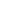, or vertical, state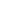. Until its polarisation is measured the photon can be in a superposition
Quantum superposition
Quantum superposition is a fundamental principle of quantum mechanics. It holds that a physical system exists in all its particular, theoretically possible states simultaneously; but, when measured, it gives a result corresponding to only one of the possible configurations.Mathematically, it...

of both these states, so its wavefunction
Wavefunction
Not to be confused with the related concept of the Wave equationA wave function or wavefunction is a probability amplitude in quantum mechanics describing the quantum state of a particle and how it behaves. Typically, its values are complex numbers and, for a single particle, it is a function of...

,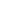, would be written: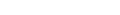The probability amplitudes of statesandare α and β respectively. When the photon's polarisation is measured, it has probabilityof being horizontally polarised, and probability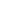of being vertically polarised.

Therefore, a photon with wavefunction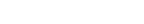whose polarisation was measured would have a probability of 1/3 to be horizontally polarised, and a probability of 2/3 to be vertically polarised.

### Normalisation

The measurement must give eitheror, so the total probability of measuringormust be 1. This leads to a constraint that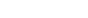; more generally the sum of the squared moduli of the probability amplitudes of all the possible states is equal to one. Wavefunctions that fulfill this constraint are called normalised wavefunctions.

### Normalisable states

The Schrödinger wave equation, describing states of quantum particles, has solutions that describe a system and determine precisely how the state changes with time. Suppose a wavefunction
Wavefunction
Not to be confused with the related concept of the Wave equationA wave function or wavefunction is a probability amplitude in quantum mechanics describing the quantum state of a particle and how it behaves. Typically, its values are complex numbers and, for a single particle, it is a function of...

is a solution of the wave equation, giving a description of the particle (position , for time ). If the wavefunction is square integrable, i.e.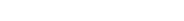for some , then is called the normalised wave function. Under the standard Copenhagen interpretation
Copenhagen interpretation
The Copenhagen interpretation is one of the earliest and most commonly taught interpretations of quantum mechanics. It holds that quantum mechanics does not yield a description of an objective reality but deals only with probabilities of observing, or measuring, various aspects of energy quanta,...

, the normalised wavefunction gives probability amplitudes for the position of the particle. Hence, at a given time , is the probability density function
Probability density function
In probability theory, a probability density function , or density of a continuous random variable is a function that describes the relative likelihood for this random variable to occur at a given point. The probability for the random variable to fall within a particular region is given by the...

of the particle's position. Thus the probability that the particle is in the volume at is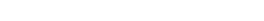Note that if any solution to the wave equation is normalisable at some time , then the defined above is always normalised, so that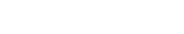is always a probability density function for all . This is key to understanding the importance of this interpretation, because for a given initial , the Schrödinger equation fully determines subsequent wavefunction, and the above then gives the probable location of the particle at all subsequent times.

### Non-normalisable states

Probability amplitudes which are not square integrable are usually interpreted as the limit of a series of functions which are square integrable. For example, the plane wave solution to the wave equation is not normalisable, so it is not possible to give a physical interpretation of it for a single particle. Instead, one way to interpret this solution is as an infinite stream of monochromatic (identical) particles, in this case the limit of the series giving the wavefunction for increasingly many particles. The definition of given above is still valid, however since now many particles are involved, there could therefore be a high probability everywhere of finding a particle nearby.

### Conservation relationship between probability amplitudes and probabilities

For more details on this topic and the proof, see probability current
Probability current
In quantum mechanics, the probability current is a mathematical quantity describing the flow of probability density. Intuitively; if one pictures the probability density as an inhomogeneous fluid, then the probability current is the rate of flow of this fluid...

.

Intuitively, since a normalised wave function stays normalised while evolving according to the wave equation, there will be a relation between the change in the probability density of the particle's position and the change in the amplitude at these positions.

Define the probability current
Probability current
In quantum mechanics, the probability current is a mathematical quantity describing the flow of probability density. Intuitively; if one pictures the probability density as an inhomogeneous fluid, then the probability current is the rate of flow of this fluid...

(or flux) as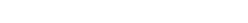measured in units of (probability)/(area × time).

Then the current satisfies the quantum continuity equation
Continuity equation
A continuity equation in physics is a differential equation that describes the transport of a conserved quantity. Since mass, energy, momentum, electric charge and other natural quantities are conserved under their respective appropriate conditions, a variety of physical phenomena may be described...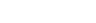## Discrete amplitudes

While the wave function describes the state of a system for the continuous variable position, there are also many discrete variables to which probabilities may also be attached, which in quantum mechanics are found from complex amplitudes.

### Example: One-dimensional quantum tunnelling

For more details on this example, see finite potential barrier.

In the one-dimensional case of particles with energy less than in the square potential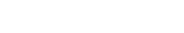the steady-state solutions to the wave equation have the form (for some constants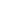)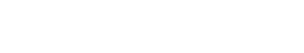The standard interpretation of this is as a stream of particles being fired at the step from the left (the direction of negative ): setting corresponds to firing particles singly; the terms containing , , and signify motion to the right, while , , and to the left. Under this beam interpretation, put since no particles are coming from the right. By applying continuity of wave functions and their derivatives at the boundaries, it is hence possible to determine the constants above.

The conclusion is that the complex value is a probability amplitude, with a real interpretation in the problem. The corresponding probability describes the probability of a particle fired from the left being reflected by the potential barrier. Note that, very neatly, just as expected.

## Probability frequency

A discrete probability amplitude may be considered as a fundamental frequency
Fundamental frequency
The fundamental frequency, often referred to simply as the fundamental and abbreviated f0, is defined as the lowest frequency of a periodic waveform. In terms of a superposition of sinusoids The fundamental frequency, often referred to simply as the fundamental and abbreviated f0, is defined as the...

in the Probability Frequency domain (spherical harmonics
Spherical harmonics
In mathematics, spherical harmonics are the angular portion of a set of solutions to Laplace's equation. Represented in a system of spherical coordinates, Laplace's spherical harmonics Y_\ell^m are a specific set of spherical harmonics that forms an orthogonal system, first introduced by Pierre...

) for the purposes of simplifying M-theory
M-theory
In theoretical physics, M-theory is an extension of string theory in which 11 dimensions are identified. Because the dimensionality exceeds that of superstring theories in 10 dimensions, proponents believe that the 11-dimensional theory unites all five string theories...

transformation calculations.

• Double-slit experiment
Double-slit experiment
The double-slit experiment, sometimes called Young's experiment, is a demonstration that matter and energy can display characteristics of both waves and particles...

• Electron shell
Electron shell
An electron shell may be thought of as an orbit followed by electrons around an atom's nucleus. The closest shell to the nucleus is called the "1 shell" , followed by the "2 shell" , then the "3 shell" , and so on further and further from the nucleus. The shell letters K,L,M,.....

• Normal distribution
• Quantum chemistry
Quantum chemistry
Quantum chemistry is a branch of chemistry whose primary focus is the application of quantum mechanics in physical models and experiments of chemical systems...

• Resonance (particle physics)
Resonance (particle physics)
In particle physics, a resonance is the peak located around a certain energy found in differential cross sections of scattering experiments. These peaks are associated with subatomic particles and their excitations...

• Uncertainty principle
Uncertainty principle
In quantum mechanics, the Heisenberg uncertainty principle states a fundamental limit on the accuracy with which certain pairs of physical properties of a particle, such as position and momentum, can be simultaneously known...

• Wave packet
Wave packet
In physics, a wave packet is a short "burst" or "envelope" of wave action that travels as a unit. A wave packet can be analyzed into, or can be synthesized from, an infinite set of component sinusoidal waves of different wavenumbers, with phases and amplitudes such that they interfere...